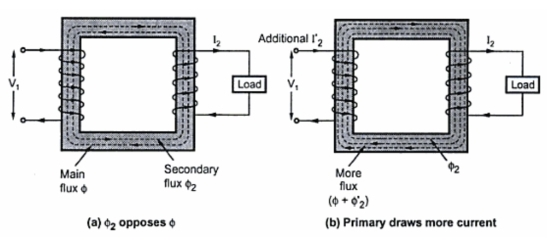When the transformer is loaded, the current I2 flows through the secondary winding. The magnetic and phase of  I2 is determined by the load. If load is inductive, I2 lags V2. If load is capacitive,  I2 leads V2 while for resistive load, I2 is in phase withV2.
There exists a secondary m.m.f. N2 I2 due to which secondary current sets up its own flux Φ2. This flux opposes the main flux Φ which is produced in the core due to magnetising component of no load current. Hence the m.m.f. is N2 I2 called demagnetising ampere-turns. This is shown in the Fig.1(a).
The flux Φ2 momentarily reduces the main flux Φ, due to which the primary induced e.m.f. also E1 reduces.This additional current drawn by primary is due to the load hence called load component of primary current denoted as I2' as shown in the Fig.1(b).Fig. 1 Transformer on load
This current I2' is in antiphase with I2. The current sets up its own flux Φ2' which opposes the flux Φ2 and helps the main fluxΦ. This flux Φ2' neutralises the flux Φ2 produced by I2. The m.m.f. i.e. ampere turns N2 I2 balances the ampere turns N2 I2. Hence the net flux in the core is again maintained at constant level.
Key point : Thus for any load condition, no load to full load the flux in the core is practically constant.
The load component current I2' always neutralises the changes in the loads. Hence the transformer is called constant flux machine.
As the ampere turns are balanced we can write,
N2 I2=N2 I2'
..                    I2' =(N2/N1) = K I2               ..................(1)
Thus when transformer is loaded, the primary current I1 has two components :
1. The no load current Io which lags V1 by angle Φo. It has two components  Im and Ic.
2. The load component I2' which in antiphase with I2. And phase of I2 is decided by the load.
Hence primary current I1 is vector sum of Io and I2'.
..                  Ī1 = Īo + Ī2                                ...............(2)
Assume inductive load, I2 lags E2 by Φ2, the phasor diagram is shown in the Fig. 2(a).
Assume purely resistive load, I2 in phase with E2, the phasor diagram is shown in the Fig.2(b).
Assume capacitive load, I2 leads E2 by Φ2, the phasor diagram is shown in the Fig. 2(c).
Note that I2' is always in antiphase with I2.Fig. 2
Actually the phase of I2 is with respect to V i.e. angle Φ2 is angle between I2 and V2. For the ideal case, E2  is assumed equal to V neglecting various drops.
The current ratio can be verified from this discussion. As the no load current Io is very small, neglecting Io we can write,
I~ I2'
Balancing the ampere turns,
NI= N1 I1 = NI
..              N2 /N1 = I1 /I= K
Under full load conditions when Io is very small compared to full load currents, the ratio of primary and secondary current is constant.
Example : A 400/200 V transformer takes 1 A at a power factor of 0.4 on no load. If the secondary supplies a load current of  50 A at 0.8 lagging power factor, calculate the primary current.
Solution : The given values are
I= 1 A, cos Φo = 0.4, I2 = 50 A and cos Φ2 = .08
K = E2/E1 = 200/400 = 0.5
...          I2' = K I2 = 0.5 x 50 = 25 A
The angle of I2' is to be decided from cos Φ2 = 0.8
Now      cos Φ2 = 0.8
...           Φ2 = 36.86o
I2' is antiphase with I2 which lags E2  by  36.86o
Consider the phasor diagram shown in the Fig. 3. The flux Φ is the reference.

Now        cos Φo = 0.4
...            Φo = 66.42o
Ī1 = Ī2'+ Īo                     ............ vector sum
Resolve Io and I2' into two components, along reference Φ and in quadrature with Φ in phase with V1.
x component of  Io = Io sin Φo = 0.9165 A
y component Io = Io cos Φo = 0.4 A
...                          Īo = 0.9165 + j 0.4 A
x component of  I2' = I2'sin Φ2 = 25 sin (36.86o ) = 15 A
y component of I2' = I2'cos Φ2 = 25 x 0.8 = 20 A
...                              I2' = 15 + j 20 A
Ī1 = 0.9165 + j 0.4 + 15 + j  20 = 15.9165 + j 20.4 A
Thus the two components of I1 are as shown in the Fig.3(c).

...           I1 = √((15.9165)2 + (20.4)2) = 25.874 A
This is the primary current magnitude.
While    tan Φ1 = 15.9165/20.4
...               Φ1 = 37.96o
Hence the primary power factor is,
cos Φ1 = cos (37.96o ) = 0.788 lagging
Key point : Remember that Φ1 is angle between V1 and I1 and as V1 is vertical, Φ1 is measured with respect to V1. So do not convert rectangular to polar as it gives angle with respect to x-axis and we want it with respect to y-axis.